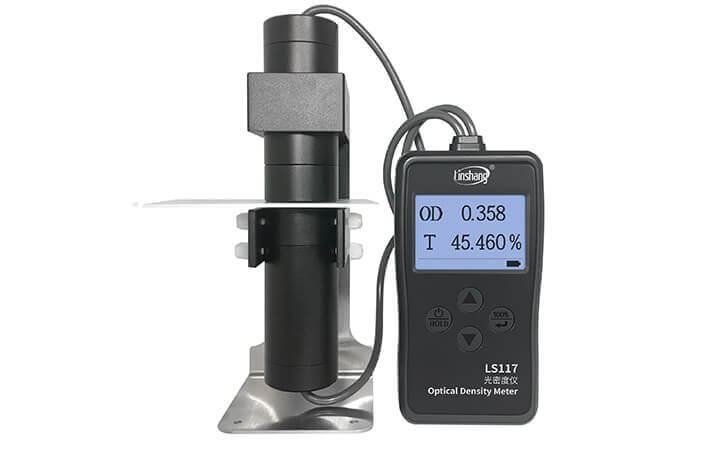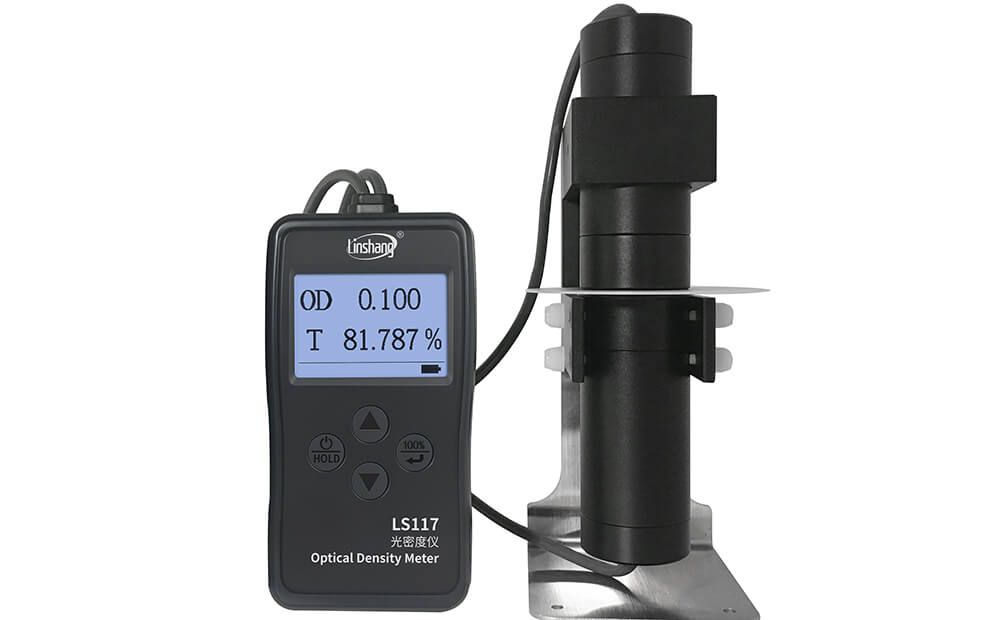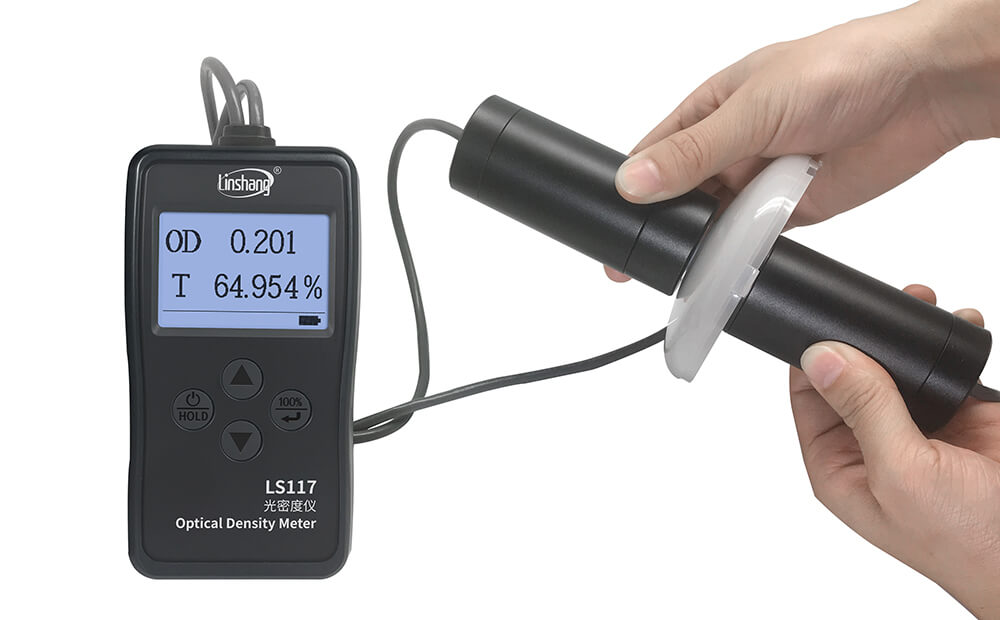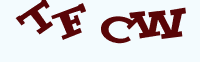# The Definition of Optical Density and the Measurement

Time:2019/03/29 10:47:00 Browse:602

Optical density is the natural logarithm of the ratio of incident to transmitted radiant power through a material. For measuring the optical density of some materials, you can use Linshang LS117 Optical Density Meter.

## What is the meaning of optical density?

In chemistry, absorbance or decadic absorbance is the common logarithm of the ratio of incident to transmitted radiant power through a material, and spectral absorbance or spectral decadic absorbance is the common logarithm of the ratio of incident to transmitted spectral radiant power through a material. Absorbance is dimensionless, and in particular is not a length, though it is a monotonically increasing function of path length, and approaches zero as the path length approaches zero. The use of the term "optical density" for absorbance is discouraged.
In physics, a closely related quantity called "optical depth" is used instead of absorbance: the natural logarithm of the ratio of incident to transmitted radiant power through a material. The optical depth equals the absorbance times ln(10).

The calculation formula of optical density is:

OD(Optical Density) = log10 (1 / Transmittance) = log10 (Incident Light / Transmitted Light)

The term absorption refers to the physical process of absorbing light, while absorbance does not always measure absorption: it measures attenuation (of transmitted radiant power). Attenuation can be caused by absorption, but also reflection, scattering, and other physical processes.## How to measure optical density values?

The operation of the optical density meter LS117 is very simple. It can be tested after the power is turned on. The OD displayed on the interface is the optical density value. T is the transmittance value.

### Measurement mode

Linshang LS117 Optical Density Meter has two measurement mode:

1. Fast mode: the measurement time is 1.1s each time. If the optical density is less than OD5, the FAST mode is recommended.

2. Slow mode: the measurement time is 2.8s each time. If the optical density is larger than OD5, the SLOW mode is recommended.

### Measurement process

1) Light transmittance test for diffuse transmission material:

As for light transmittance of various milky, fogged, abrasive and matte materials, such as milky and abrasive glasses, ceiling lamps, diffuser, and zirconium oxide, etc. The two testing methods are adopted subject to size of the test sample.

Testing of small samples

Tight the light source probe with the receiving probe on the holder, the light transmittance is displayed as 100.00% in the interface. Lift up the receiving probe and put the test sample into the holder, and then tightly attach the receiving probe to the light source probe again. The current “T” value is the transmittance value of the test sample, and the current OD value is the optical density value of the test sample.Optical density meter test small samples

Testing of large samples

In the case that it's impossible for the test sample (like large lamp chimney) to be put into the holder and it's necessary to remove the light source probe and the receiving probe from the holder, the Probe-in-hands method is used to measure the sample, with the testing process as shown in the followings:

• Align and keep those two probes in tight before powering the meter on. After “Warm-up”, the meter enters into measurement interface, with T displayed as 100.00%.

• Separate two probes and clamp the test sample. The current T value is the light transmittance value of the test sample, and the current OD value is the optical density value of the test sample (it's necessary to match two test probes together and make convex surface of the object face to the receiving probe).Optical density meter test larger samples

2) Optical density measurement of films

In the case that there is no sample and the light source probe is tightly attached to the receiving probe, when OD in the interface is displayed as 0.000, the measurement begins.

Lift up the receiving probe and put into the test sample, and then tightly attach the receiving probe to the light source probe again. The current OD value is the optical density value of the tested film.

## What materials can an Optical Density Meter measure?

Linshang LS117  Optical Density Meter is used to measure the transmittance and optical density of the materials, which mainly include the following three kinds of materials:

• The light transmittance of various diffuse transmission materials such as milky, fogged, abrasive, and matte materials.

• Absolute optical density of materials like X-ray film, and aluminum film.

• The absolute optical density measurement of all films.

Enquiry

### EnquiryClick image refresh captcha
x